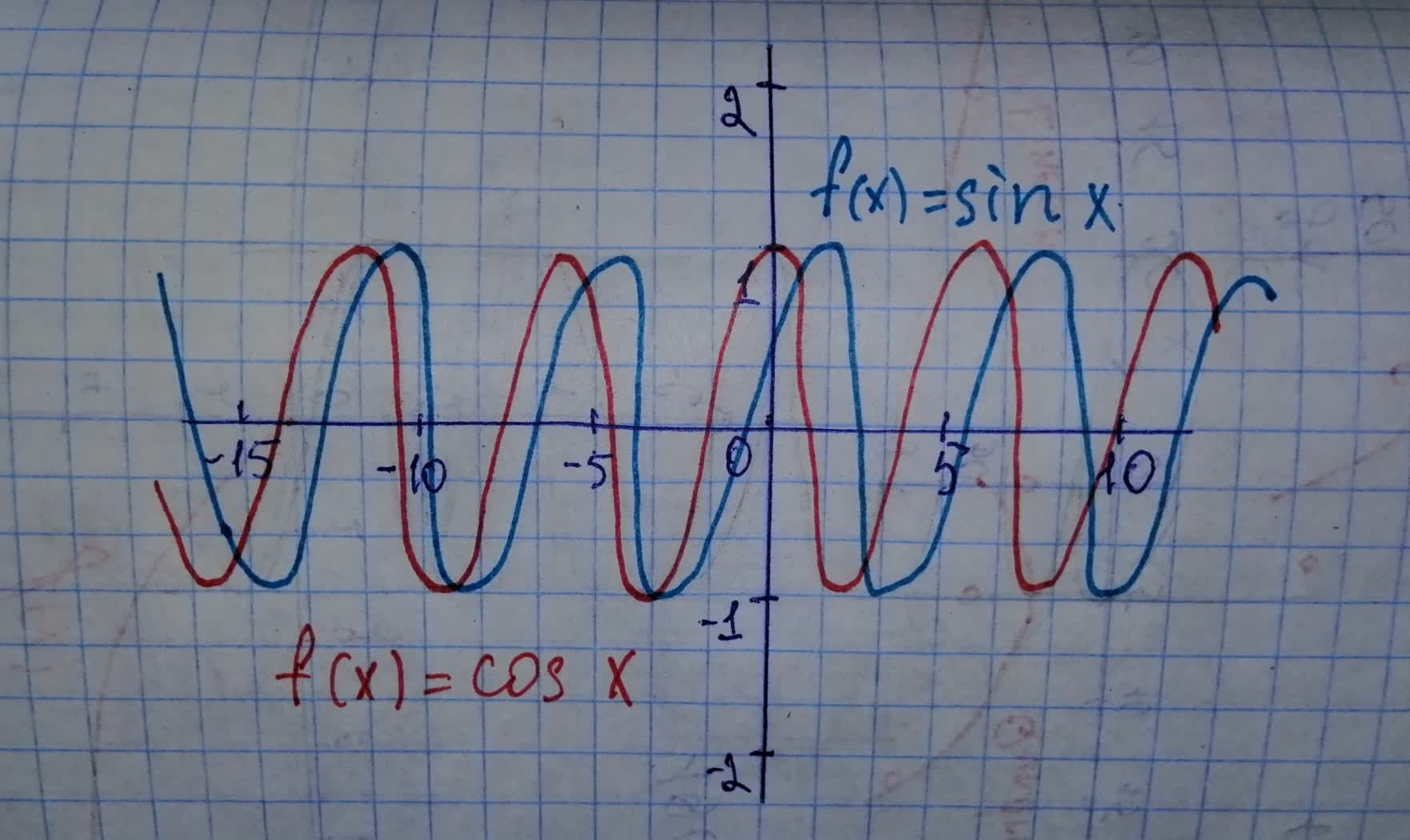Question# Describe any similarities and differences. Refer to the end behaviour, local maximum and local minimum points, and maximum and minimum points. a) Sketch graphs of y=sin x and y=cos x. b) Compare the graph of a periodic function to the graph of a polynomial function.

Polynomial graphs
ANSWEREDDescribe any similarities and differences. Refer to the end behaviour, local maximum and local minimum points, and maximum and minimum points.
a) Sketch graphs of $$\displaystyle{y}={\sin{\ }}{x}$$ and $$\displaystyle{y}={\cos{\ }}{x}.$$
b) Compare the graph of a periodic function to the graph of a polynomial function.2021-02-21
Step 1
a)Step 2
b) Periodic function: A function with a graph that repeats identically smoothly from left to right. It can be represented by $$\displaystyle{f{{\left({x}\right)}}}={f{{\left({x}\ +\ {p}\right)}}}{>}$$
There is no the end points for the periodic functions and have uncountable number from the turning points (local maximum and local minimum points).
The graphs of polynomial functions are continuous, and smooth graphs.
The turning points and the behavior of polynomial function graphs depend mainly on its degree.